# 1 Introduction

Spatial analysis of the urban environment frequently requires estimation of shading. For example -

• The amount of time a given point / roof / facade is shaded may determine the utility of installing Photo-Voltaic cells for electricity production

• The Sky View Factor (SVF) of a given street is associated with its microclimate

• Calculating shadow footprint on a green park helps urban planners provide guidelines for maximal height of a new building

These types of calculations (and more advanced ones) are usually restricted to proprietary software dealing with 3D models, such as ESRI’s ArcScene. Terrain-based solutions (i.e. Digital Elevation Model, DEM) are more common, in both open-source (GRASS GIS) as well as proprietary (ArcGIS) software. The insol R package (Corripio 2014) provides such capabilities in R. However terrain-based approaches may not be appropriate for an urban environment, for two reasons. First, a continuous elevation surface at the necessary resolution for the urban context (e.g. LIDAR) may not be available and is expensive to produce. Second, the DEMs cannot adequately represent individual urban elements such as building facades, thus limiting the interpretability of results. The shadow package aims at addressing these limitations. The shadow package operates on a vector layer of building outlines along with their heights, rather than a DEM. Such data are generally much more available, either from local municipalities or from global datasets such as OpenStreetMap. Therefore the resulting shadow estimates correspond to urban environment such as individual buildings or facades. It should be noted that the approach assumes a flat terrain and no obstacles (e.g. trees) other than the buildings, which may be inappropriate in certain situations (e.g. a mountainous urban area).

## 1.1 Calculation

The functions currently included shadow are based on the trigonometric relations in the triangle defined by the sun rays, the ground (or plane parallel to the ground) and an obstacle. For example, as shown in Figure 1.1, shadow height ($$h_{shade}$$) at a given location ($$observer$$) can be calculated based on the sun elevation angle ($$\alpha$$) in case there is one obstacle with a known height ($$h_{build}$$) and at a known distance ($$dist$$) -

$$h_{shade} = h_{build} - dist \cdot tan(\alpha)$$

This approach can be expanded for the general case of multiple obstacles, in which case we must take the maximum value of all potential shadow heights caused by the obstacles.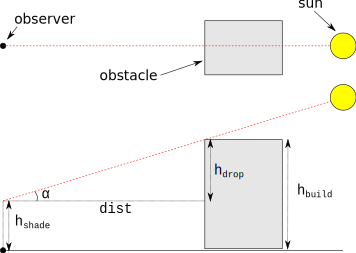Figure 1.1: Shade height calculation

# 2 Functions

The shadow package currently contains three main functions, as well as several helper functions. The main functions give three distinct aspects of shading -

• The shadowHeight function calculates shadow heights.

• The shadowFootprint function calculates the shadow footprint on the ground.

• The SVF function calculates the Sky View Factor (SVF), which is a measure of sky proportion not obstructed to the viewer.

# 3 Examples

Before going into the examples, we load the shadow package as well as packages sp (loaded automatically), raster, rgeos and rgdal -

library(shadow)
library(raster)
library(rgeos)

In the examples we will use a polygonal layer representing four buildings (rishon) along having a height attribute (BLDG_HT) as shown on Figure 3.1 -

plot(rishon)
text(gCentroid(rishon, byid = TRUE), rishon$BLDG_HT)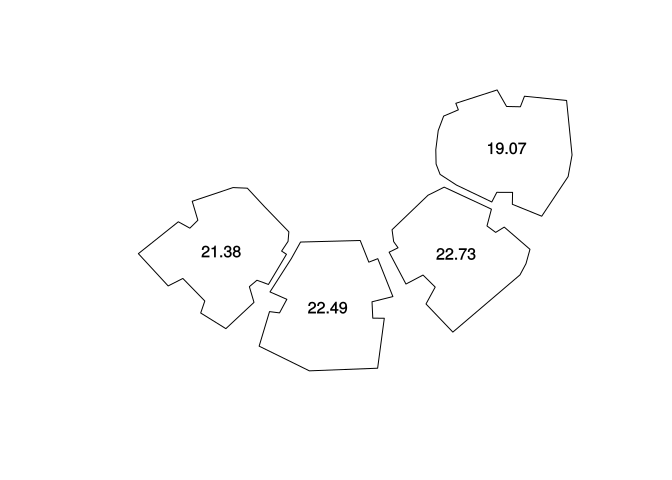Figure 3.1: Sample buildings and heights ## 3.1 Shadow height The shadowHeight function calculates shadow heights at given locations and sun positions. For example, to calculate shade height at the centroid of the layer (location), on 2004-12-24 13:30:00 we first need to determine the sun elevation and azimuth at that time. This can be done with function solarpos from package maptools - location = gCentroid(rishon) time = as.POSIXct("2004-12-24 13:30:00", tz = "Asia/Jerusalem") location_geo = spTransform(location, "+proj=longlat +datum=WGS84") solar_pos = maptools::solarpos(location_geo, time) solar_pos #> [,1] [,2] #> [1,] 208.7333 28.79944 Now we know the sun azimuth (208.7) and elevation (28.8). Given sun position, the layer of obstacles and queried location, shade height can be calculated with shadowHeight - h = shadowHeight( location = location, obstacles = rishon, obstacles_height_field = "BLDG_HT", solar_pos = solar_pos ) h #> [,1] #> [1,] 19.86451 Shade height at the queried point is 19.86 meters. Note the warning regarding the units of the BLDG_HT attribute. The function has no way of knowing that the height attribute units are the same as those of the Coordinate Reference System (CRS) spatial distance units. It is up to the user to make sure they are in agreement. The following code and subsequent Figure 3.2 illustrate how the calculation is carried out ‘behind the scenes’. First, a line of sight ray is drawn between the point of interest location and the sun position based on its azimuth sun_az. Potential intersections inter are then detected. Finally, the shadow height induced by each intersection is calculated based on the distance to intersection, sun elevation sun_elev and building height. The final result (19.86 meters) is the maximum value of these potential heights. sun = shadow:::.sunLocation( location = location, sun_az = solar_pos[1, 1], sun_elev = solar_pos[1, 2] ) sun_ray = ray(from = location, to = sun) build_outline = as(rishon, "SpatialLinesDataFrame") inter = gIntersection(build_outline, sun_ray) plot(rishon) text(gCentroid(rishon, byid = TRUE), rishon$BLDG_HT)
plot(location, add = TRUE)
text(location, round(h, 2), pos = 3)
plot(sun_ray, add = TRUE, col = "yellow")
plot(inter, add = TRUE, col = "red")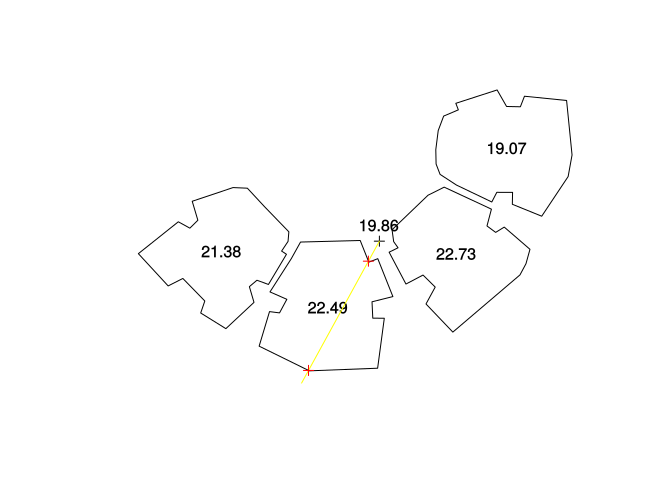Figure 3.2: Shade height at a single location

The shadowHeight function also accepts Raster objects, in which case the calculation is repeated for all pixels and a continuous shadow height surface is returned.

First, we will a RasterLayer covering the examined area with a spatial resolution of 2 meters -

ext = as(extent(rishon) + 50, "SpatialPolygons")
r = raster(ext, res = 2)
proj4string(r) = proj4string(rishon)

Replacing location with the raster r we now can calculate a shadow height surface -

r = shadowHeight(
location = r,
obstacles = rishon,
obstacles_height_field = "BLDG_HT",
solar_pos = solar_pos
)

The resulting grid can plotted with following code section (Figure 3.3). Note the partial shade on the roof of the 19.07-m building which is caused by the slightly taller 22.73-m building.

plot(r, col = grey(seq(0.9, 0.2, -0.01)))
contour(r, add = TRUE)
plot(rishon, add = TRUE, border = "red")
text(gCentroid(rishon, byid = TRUE), rishon$BLDG_HT)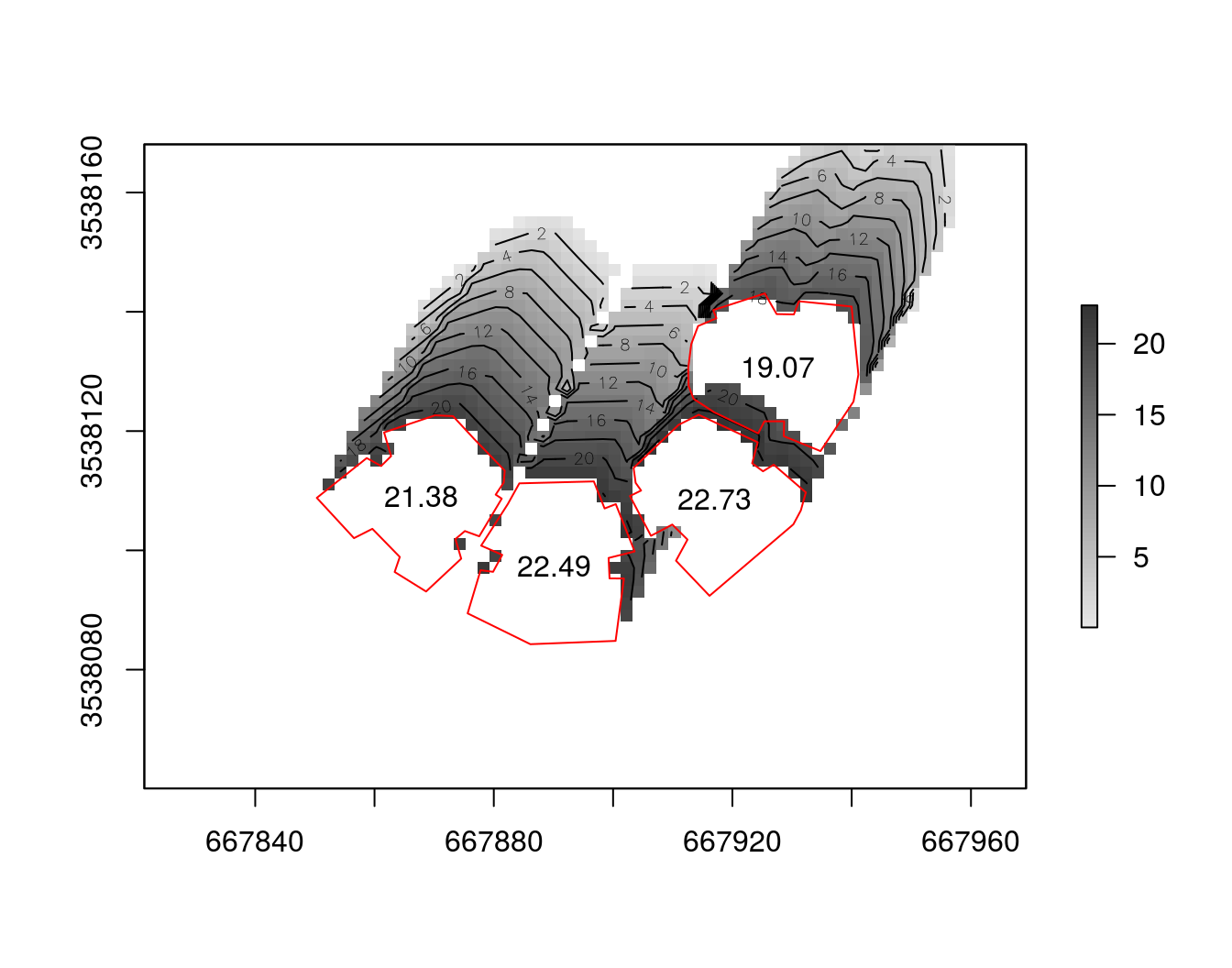Figure 3.3: Shade height (m) grid ## 3.2 Shadow footprint The shadowFootprint function calculates the geometry of shadow projection on the ground, rather than its height at discrete sampling points. The resulting layer can be used to calculate the proportion of shaded surface out of a defined area, to examine which building shades a given element, etc. For example, suppose a playground is being built in vicinity to the four buildings in rishon, as shown on Figure 3.4. park_location = raster::shift(location, y = 20, x = -8) park = rgeos::gBuffer(park_location, width = 12) plot(rishon) text(gCentroid(rishon, byid = TRUE), rishon$BLDG_HT)
plot(park, col = "lightgreen", add = TRUE)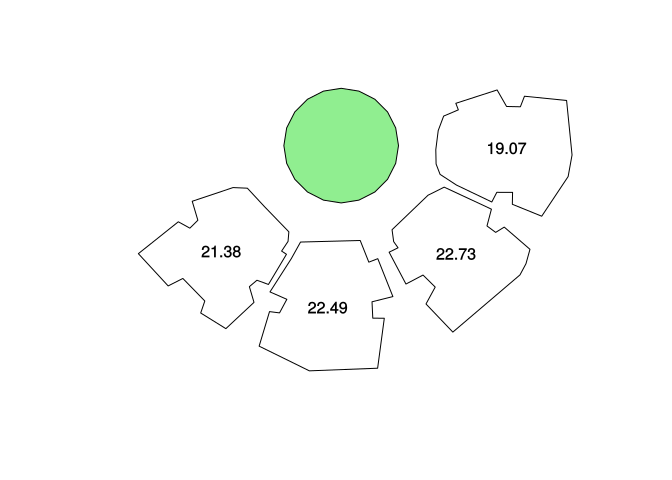Figure 3.4: Park location

Using shadowFootprint we can determine to what extent the palyground is shaded at a given time. For example, sun position at 2004-06-24 09:30:00 is (88.8, 46.7). The corresponding park shade proportion can be calculated by intersecting the shade footprint with park area and calculating the ratio. The result is 34.5%.

time2 = as.POSIXct("2004-06-24 09:30:00", tz = "Asia/Jerusalem")
solar_pos2 = maptools::solarpos(location_geo, time2)
solar_pos2
#>          [,1]   [,2]
#> [1,] 88.83113 46.724
obstacles = rishon,
obstacles_height_field = "BLDG_HT",
solar_pos = solar_pos2
)
park_shade = gIntersection(park, footprint)
#>  0.3447709

The following code section graphically demonstrates the resulting shaded proportion (Figure 3.5).

plot(footprint,  col = adjustcolor("lightgrey", alpha.f = 0.5))
plot(rishon, col = "darkgrey", add = TRUE)
plot(park, col = "lightgreen", add = TRUE)
plot(park_shade, col = adjustcolor("darkgreen", alpha.f = 0.5), add = TRUE)
text(gCentroid(park_shade), round(shade_prop, 2))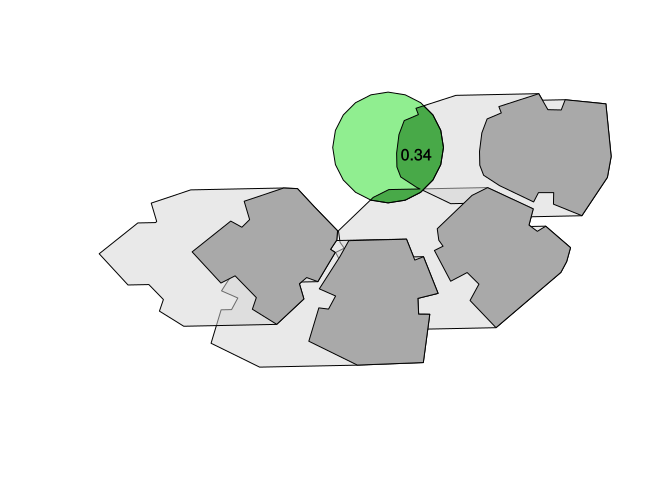Figure 3.5: Shade footprint at 2004-06-24 09:30:00

The calculation can be repeated for a sequence of times, rather than a single one, to monitor the daily (monthly, annual) course of shade proportions. For example, the following code creates a matrix solar_pos_seq which represents the sun positions course on 2004-06-24 at hourly intervals -

time_seq = seq(
from = as.POSIXct("2004-06-24 03:30:00", tz = "Asia/Jerusalem"),
to = as.POSIXct("2004-06-24 22:30:00", tz = "Asia/Jerusalem"),
by = "1 hour"
)
solar_pos_seq = maptools::solarpos(location_geo, time_seq)
solar_pos_seq
#>            [,1]       [,2]
#>  [1,]  41.08360 -21.769851
#>  [2,]  51.77580 -12.527348
#>  [3,]  60.65421  -1.806295
#>  [4,]  68.26563   9.597935
#>  [5,]  75.12424  21.620929
#>  [6,]  81.74838  34.061974
#>  [7,]  88.83113  46.723999
#>  [8,]  97.78504  59.410673
#>  [9,] 113.28070  71.684791
#> [10,] 160.14772  80.943168
#> [11,] 233.16186  76.606322
#> [12,] 256.70087  64.978904
#> [13,] 267.54640  52.385195
#> [14,] 275.20092  39.682139
#> [15,] 281.92087  27.120222
#> [16,] 288.60587  14.879269
#> [17,] 295.81954   3.268622
#> [18,] 304.06676  -7.945221
#> [19,] 313.88346 -17.870062
#> [20,] 325.79830 -26.100500

Using a for loop over solar_pos_seq, the following code section calculates the vector of park shade proportions over the entire day. Note the two conditional statements: (1) shade proportion is maximal (i.e. 1) when sun is below the horizon and (2) shade proportion is minimal (i.e. 0) when no intersections are detected between the park and the shade footprint.

shade_props = rep(NA, nrow(solar_pos_seq))
for(i in 1:nrow(solar_pos_seq)) {
if(solar_pos_seq[i, 2] < 0)
shade_props[i] = 1 else {
footprint =
obstacles = rishon,
obstacles_height_field = "BLDG_HT",
solar_pos = solar_pos_seq[i, , drop = FALSE]
)
park_shade = gIntersection(park, footprint)
else
}
}

Figure 3.6 summarizes the temporal variation in park shade proportion over the chosen day. The individual value we manually calculated in a previous step (2004-06-24 09:30:00) is highlighted in red.

plot(
time_seq,
)